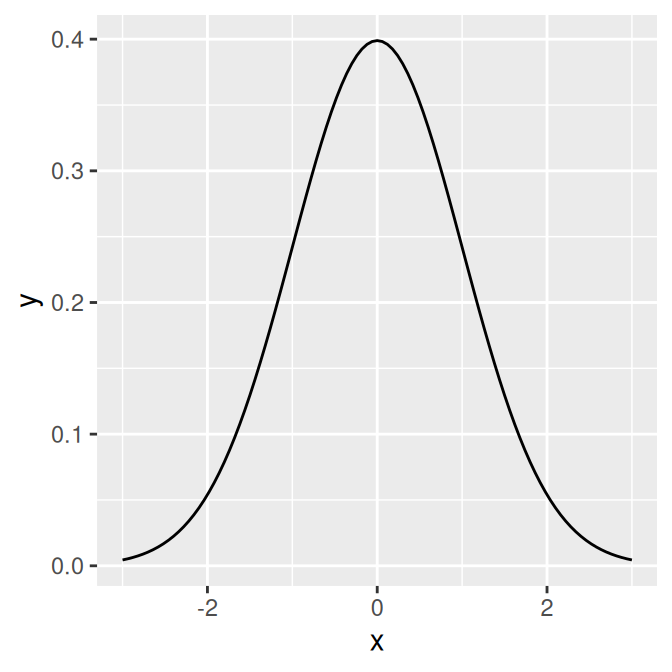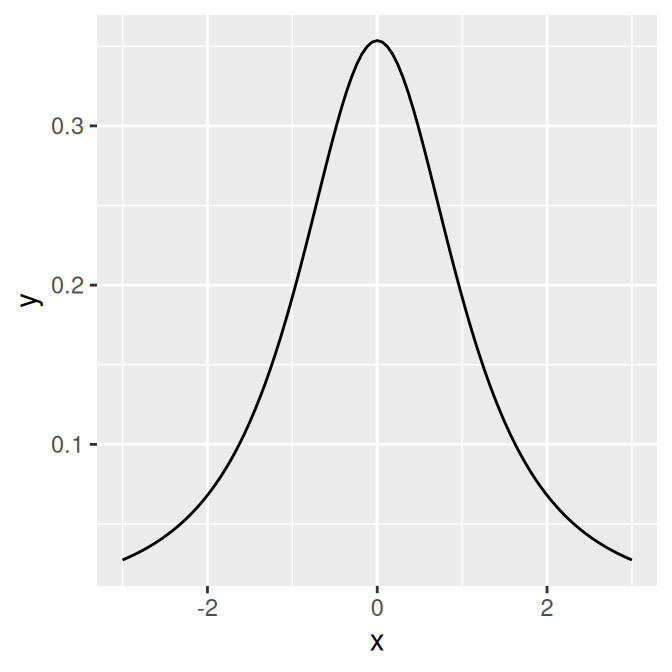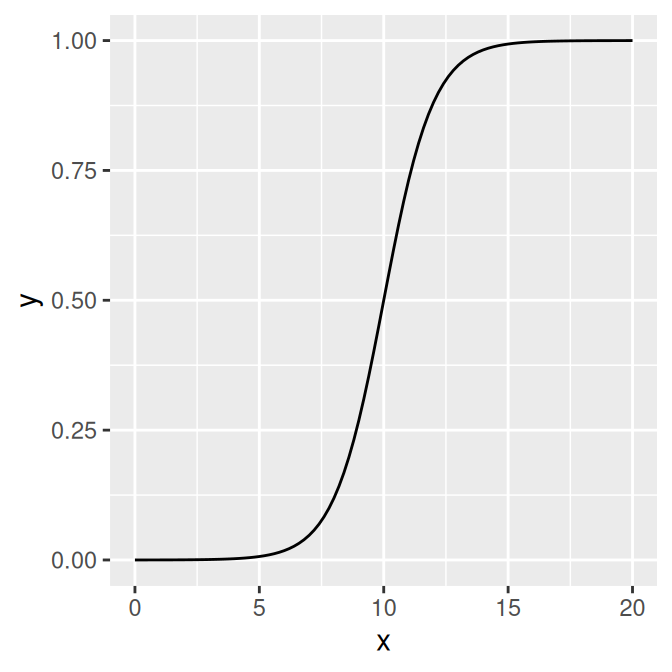## 13.2 Plotting a Function

### 13.2.1 Problem

You want to plot a function.

### 13.2.2 Solution

Use `stat_function()`. It’s also necessary to give ggplot a dummy data frame so that it will get the proper x range. In this example we’ll use `dnorm()`, which gives the density of the normal distribution (Figure 13.4, left):

``````# The data frame is only used for setting the range
p <- ggplot(data.frame(x = c(-3, 3)), aes(x = x))

p + stat_function(fun = dnorm)``````

### 13.2.3 Discussion

Some functions take additional arguments. For example, `dt()`, the function for the density of the t-distribution, takes a parameter for degrees of freedom (Figure 13.4, right). These additional arguments can be passed to the function by putting them in a list and giving the list to args:

``p + stat_function(fun = dt, args = list(df = 2))``Figure 13.4: The normal distribution (left); The t-distribution with `df=2` (right)

It’s also possible to define your own functions. It should take an x value for its first argument, and it should return a y value. In this example, we’ll define a sigmoid function (Figure 13.5):

``````myfun <- function(xvar) {
1 / (1 + exp(-xvar + 10))
}

ggplot(data.frame(x = c(0, 20)), aes(x = x)) +
stat_function(fun = myfun)``````Figure 13.5: A user-defined function

By default, the function is calculated at 101 points along the x range. If you have a rapidly fluctuating function, you may be able to see the individual segments. To smooth out the curve, pass a larger value of n to `stat_function()`, as in `stat_function(fun=myfun, n=200)`.

For plotting predicted values from model objects (such as `lm` and `glm`), see Recipe 5.7.